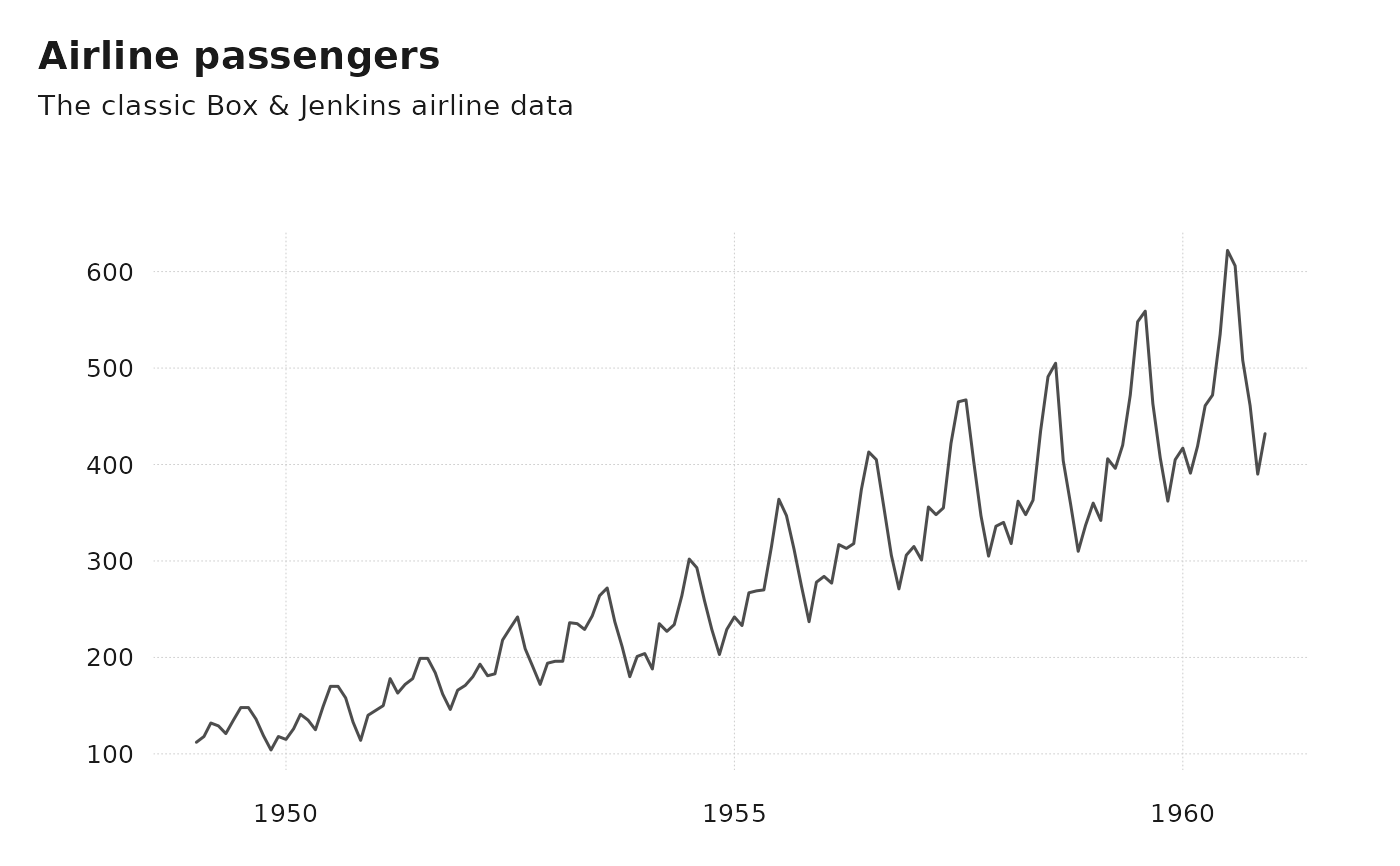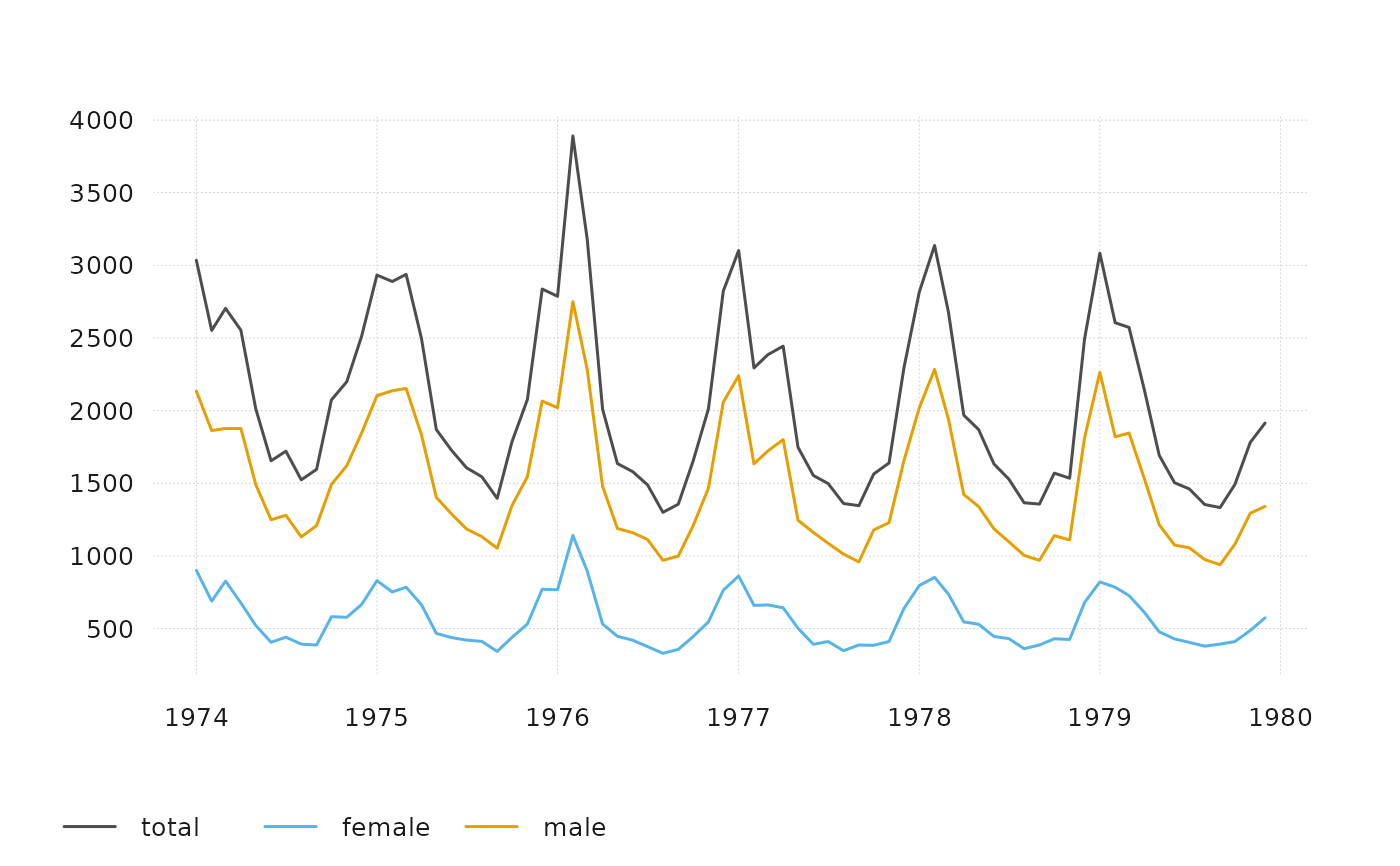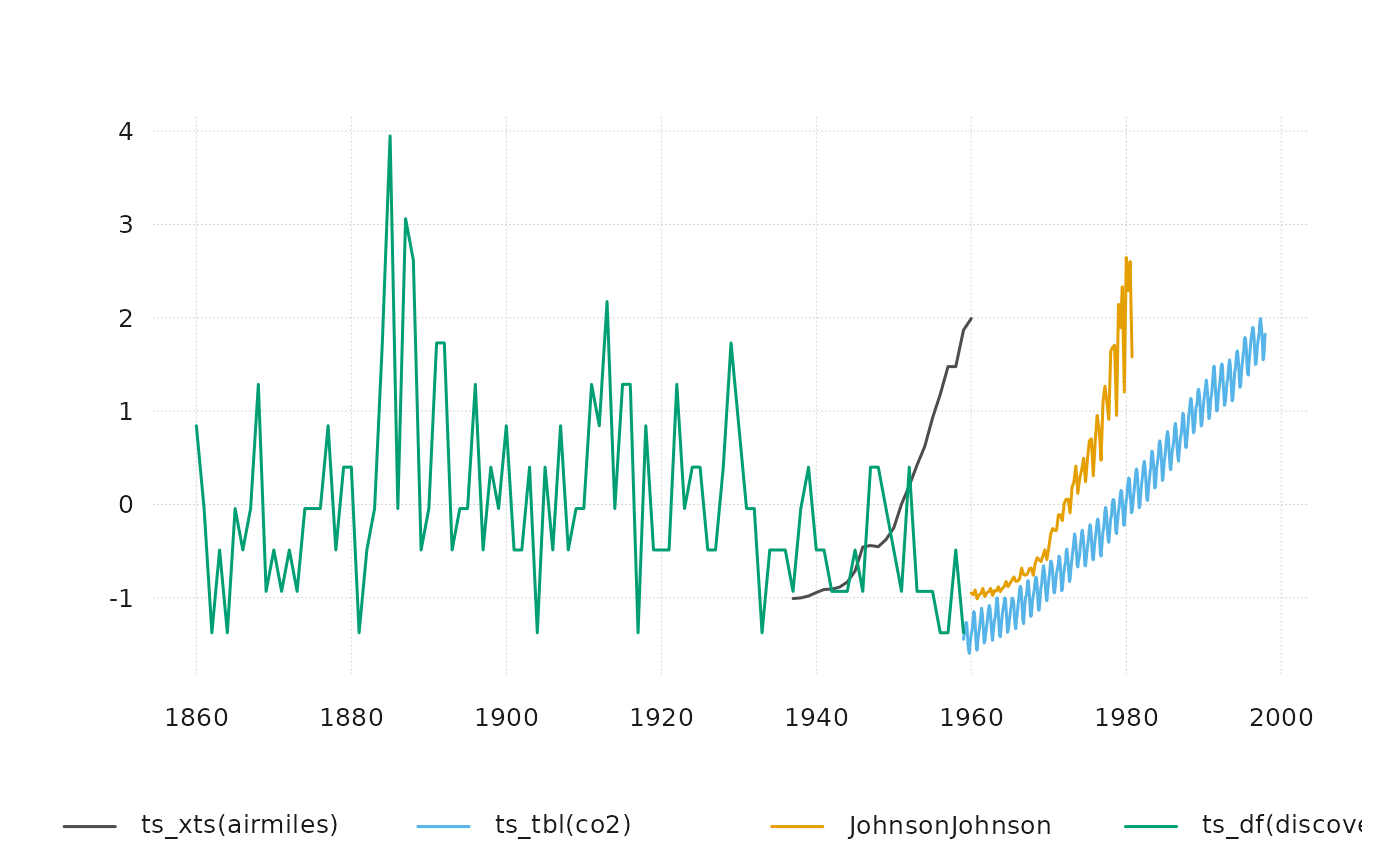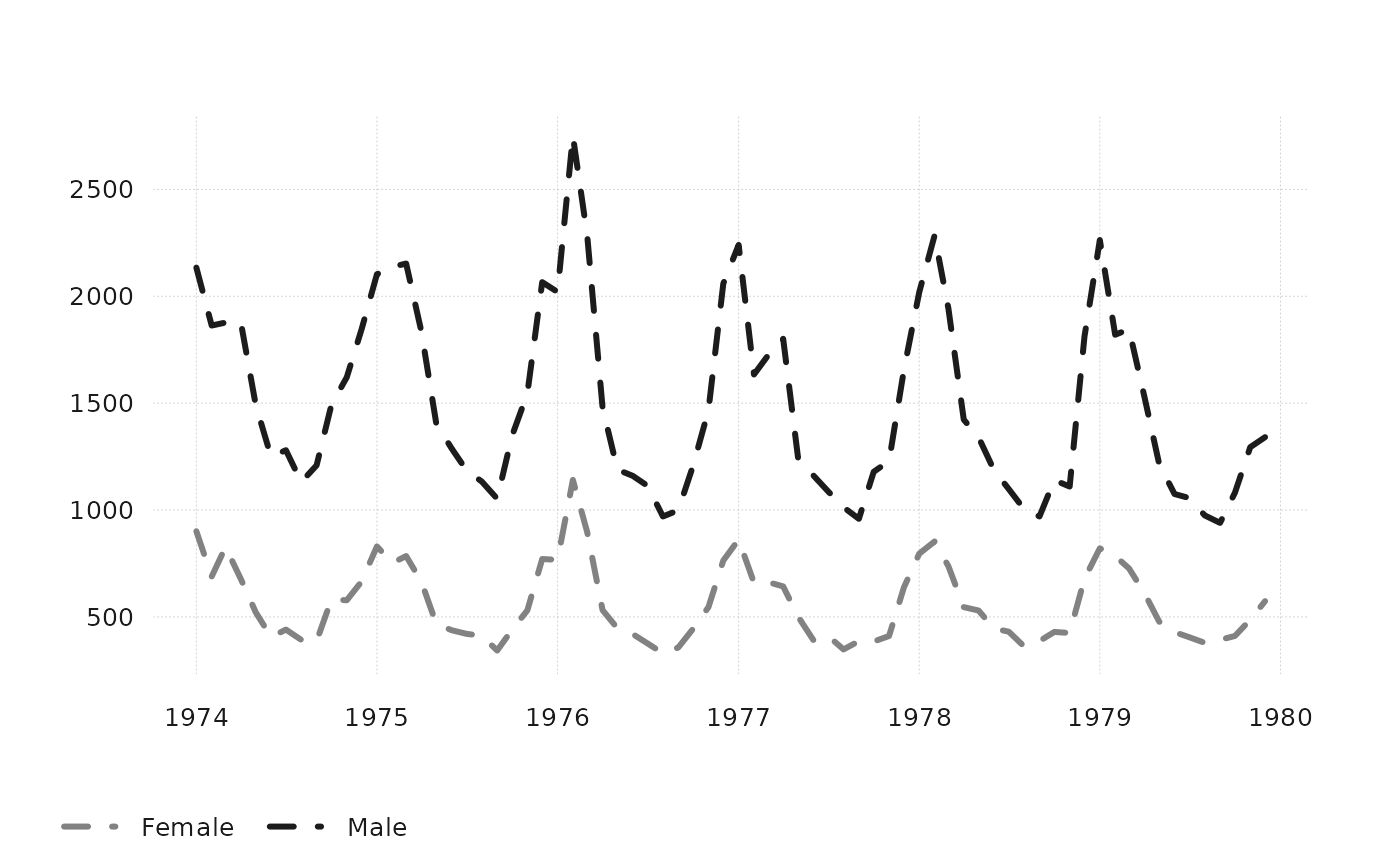`ts_plot()` is a fast and simple plotting function for ts-boxable time series, with limited customizability. For more theme options, use `ts_ggplot()`.

## Usage

``ts_plot(..., title, subtitle, ylab = "", family = getOption("ts_font", "sans"))``

## Arguments

...

ts-boxable time series, an object of class `ts`, `xts`, `zoo`, `zooreg`, `data.frame`, `data.table`, `tbl`, `tbl_ts`, `tbl_time`, `tis`, `irts` or `timeSeries`.

title

title (optional)

subtitle

subtitle (optional)

ylab

ylab (optional)

family

font family (optional, can also be set via `options`)

## Details

Both `ts_plot()` and `ts_ggplot()` combine multiple ID dimensions into a single dimension. To plot multiple dimensions in different shapes, facets, etc., use standard ggplot.

Limited customizability of `ts_plot` is available via options. See examples.

`ts_ggplot()`, for a plotting function based on ggplot2. `ts_dygraphs()`, for interactive time series plots. `ts_save()` to save a plot to the file system.

## Examples

``````# \donttest{
ts_plot(
AirPassengers,
title = "Airline passengers",
subtitle = "The classic Box & Jenkins airline data"
)# naming arguments
ts_plot(total = ldeaths, female = fdeaths, male = mdeaths)# using different ts-boxable objects
ts_plot(ts_scale(ts_c(
ts_xts(airmiles),
ts_tbl(co2),
JohnsonJohnson,
ts_df(discoveries)
)))# customize ts_plot
op <- options(
tsbox.lwd = 3,
tsbox.col = c("gray51", "gray11"),
tsbox.lty = "dashed"
)
ts_plot(
"Female" = fdeaths,
"Male" = mdeaths
)options(op) # restore defaults
# }
``````